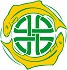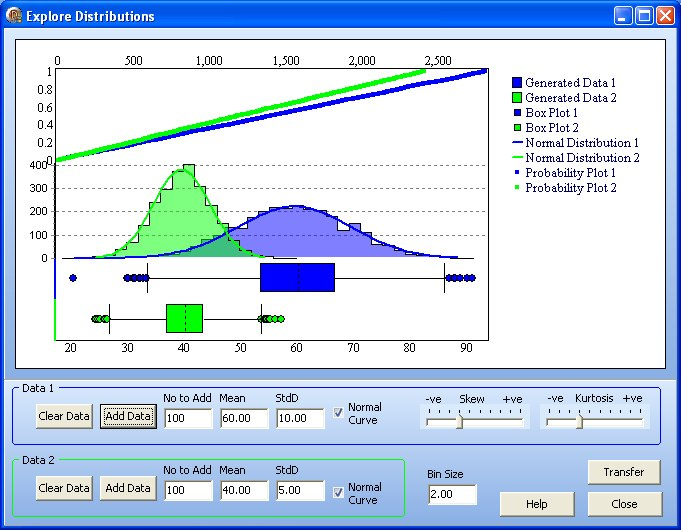Explore distributions: Learning through simulation

The Explore Distributions function provides a clear introduction to distribution:

• Create normal, skewed and kurtic distributions.
• Transfer simulated data sets to use in the main program to explore how distribution affects various analyses.
• See the effect of bin size and standard deviation on the shape of a curve.
• Combines generated data, normal distribution, probability plot and box & whisker plot to see how they inter-relate.Vividly demonstrate the different types of distribution

Create different distributions, and show how they